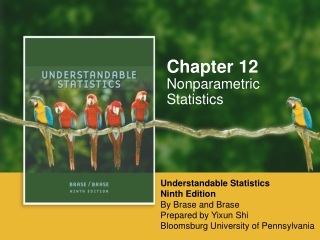DownloadDownload PresentationNonparametric Statistics

# Nonparametric Statistics

Download Presentation## Nonparametric Statistics

- - - - - - - - - - - - - - - - - - - - - - - - - - - E N D - - - - - - - - - - - - - - - - - - - - - - - - - - -
##### Presentation Transcript

1. Chapter 12 Nonparametric Statistics Understandable Statistics Ninth Edition By Brase and Brase Prepared by Yixun Shi Bloomsburg University of Pennsylvania

2. Nonparametric Situations • At times, we will not know anything about the distributions of the populations from which we are sampling. • Recall that all of our inference techniques thus far have assumed either a normal or binomial distribution from the populations of interest.

3. Nonparametric Tests • Advantages: • Easy to apply • Quite general in nature • Disadvantages: • Wastes information • Accept the null hypothesis more often than with other tests • Less sensitive

4. The Sign Test • We wish to compare two populations. • Populations are not independent

5. Sign Test Method

6. Sign Test Method

7. Sign Test Method

8. Rank-Sum Test • Data values from the two populations are not paired. • Normal assumptions are not satisfied, or are at least questionable.

9. Rank-Sum Test

10. Rank-Sum Test

11. Rank-Sum Test

12. Rank-Sum Test

13. Spearman Rank Correlation • Suppose we have a sample of size n of paired data points (x, y). • The data points, (x, y), must be ranked variables. • The Spearman Rank Correlation will tell us if the data pairs have a monotone increasing or a monotone decreasing relationship.

14. Spearman Rank Correlation Coefficient

15. Spearman Rank Correlation Properties

16. Spearman Rank Correlation Properties

17. Test for Spearman Correlation

18. Test for Spearman Correlation

19. Spearman Correlation Test

20. Spearman Correlation Test

21. Spearman Correlation Test

22. Runs Test for Randomness • Definitions:

23. Runs Test for Randomness Hypotheses

24. Conducting the Test

25. Constructing a Runs Test

26. Constructing a Runs Test

27. Constructing a Runs Test

28. Constructing a Runs Test